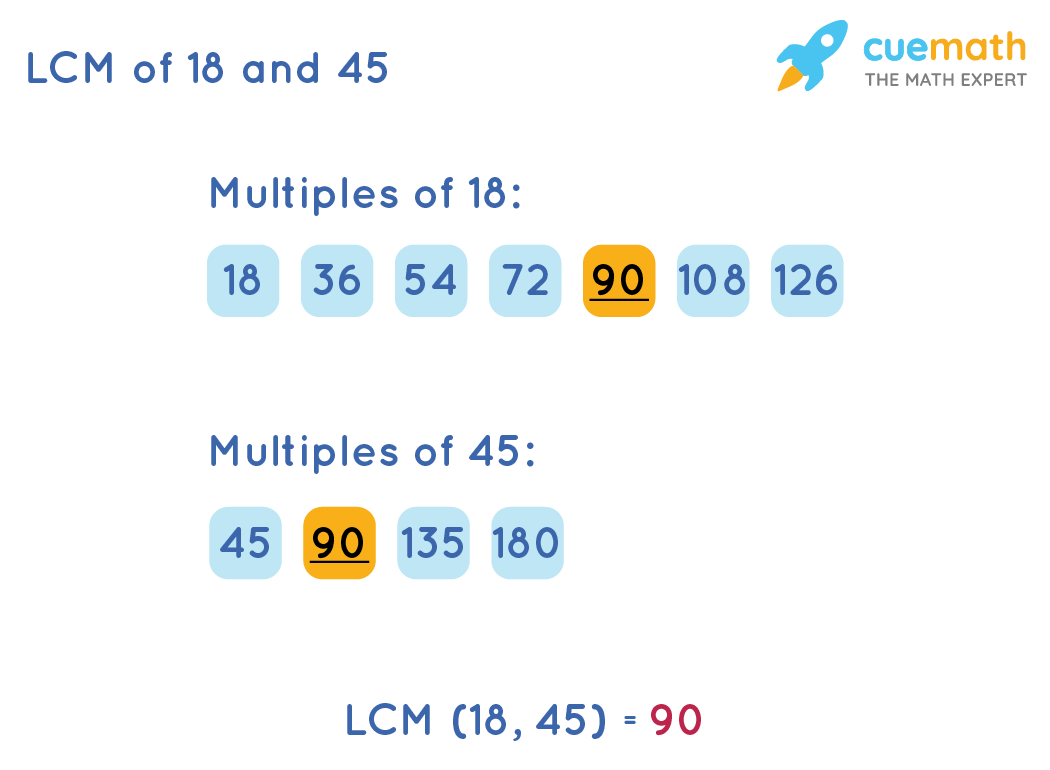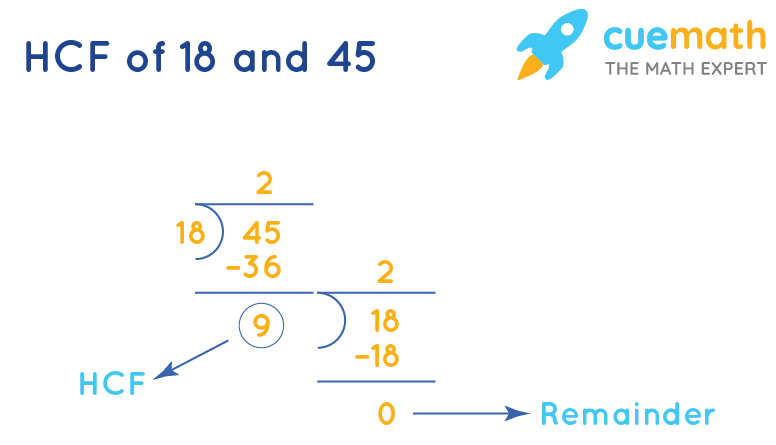# What is The HCF and LCM of 18 and 45?

## Question: What is The HCF and LCM of 18 and 45?

LCM of 18 and 45 would be the least number which is exactly divisible by both 18 and 45 and HCF (Highest Common Factor) of 18 and 45 is the greatest possible number which divides both 18 and 45 exactly.

## Explanation:

Least Common Multiple: It is the smallest number which perfectly or exactly divisible by the given numbers.

### Listing Method to get LCM of 18 and 45

• List down the first few multiples of 18 and 45
• Identify the common multiples of the two.
• The least, that is the smallest, among the common multiples, is the LCM of 18 and 45### HCF of 18 and 45 by Long Division

Follow the steps given below to find the highest common multiple of 18 and 45

Step 1: Divide 45 by 18 and check the remainder. Here, the remainder is 9.

Step 2: Make the remainder 9 as the divisor and the divisor 18 as the dividend and perform the long division again.

Step 3: Continue till you get the remainder as 0HCF of 18 and 45 is the last divisor of the division process. So, HCF of 18 and 45 is 9

You can find the HCF in any of the above methods but the solution will be the same.

### Therefore, HCF of 18 and 45 is 9 and LCM of 18 and 45 is 180.

SHEETS
GCF(HCF) - LCM Worksheet
GCF(HCF) - LCM Worksheet